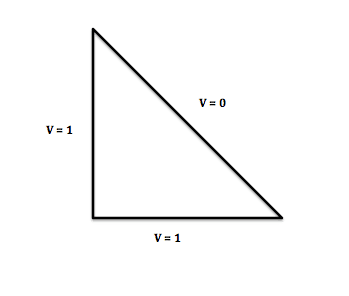# Solving Laplace's Equation for 2D isosceles right triangle

## Homework Statement

Find the two-dimensional solution to Laplace's equation inside an isosceles right triangle. The boundary conditions are as is shown in the picture:The length of the bottom and left side of the triangle are both L.

## Homework Equations

Vxx+Vyy=0
V=X(x)Y(y)
From the image, it is clear that two of the boundary conditions are...
V(0,y) = 1
V(x,0) = 1

## The Attempt at a Solution

My problem is getting started. I need more boundary conditions, but I am not sure how to get them. Also, I need use superposition or something to impose periodicity onto some part of this problem, but I can figure out how to do it. Also, another option is the zero option where X(x) = Y(y) = 0, but that doesn't seem to work either.

The only other boundary condition I can think of is V(x,L-x) = 0, but that doesn't seem to be enough information.

Can anyone help me get started with this problem? Thanks in advance.

Orodruin
Staff Emeritus
Homework Helper
Gold Member
Why do you not think you have enough boundary conditions?
Given a (reasonably nice) region, it is sufficient to know the value on the boundary or the normal derivative on the boundary in order to solve the Laplace equation.

Why do you not think you have enough boundary conditions?
Given a (reasonably nice) region, it is sufficient to know the value on the boundary or the normal derivative on the boundary in order to solve the Laplace equation.
Are my boundary conditions correct? It seems to me that either I don't have enough or perhaps the one on the diagonal is wrong because the subsequent equations for X(x), Y(y) and V don't seem to simplify enough when I plug the BC's in.

Orodruin
Staff Emeritus
Homework Helper
Gold Member
There is no guarantee that your eigenfunctions or solution will be separable in x and y. The boundary conditions are sufficient for a solution to exist.

There is no guarantee that your eigenfunctions or solution will be separable in x and y. The boundary conditions are sufficient for a solution to exist.
I'm pretty sure there is some way to find separable solutions, because the assignment is only about Laplace's equation involving separable solutions. However, I just can't seem to figure out how to apply the BC's to find the form of the solutions. This first step is what I am stuck on.

vela
Staff Emeritus
Homework Helper
There might be an easier way to do this, but here's a suggestion. Consider a square with V=1 along the left and bottom sides and V=-1 along the top and right sides. It seems plausible that you'll have V=0 along the diagonal going from the top left to bottom right, just as the problem requires, and with a square region, you'll be able to apply separation of variables in a straightforward way.

There might be an easier way to do this, but here's a suggestion. Consider a square with V=1 along the left and bottom sides and V=-1 along the top and right sides. It seems plausible that you'll have V=0 along the diagonal going from the top left to bottom right, just as the problem requires, and with a square region, you'll be able to apply separation of variables in a straightforward way.
Yes I was actually considering that, but I wasn't completely sure if I was justified in thinking the diagonal would be zero. You suggesting it gives me more confidence in it, so I'll try that route. Thanks.

Orodruin
Staff Emeritus